##全国校区# R语言描述统计第二弹 | 不同舱位乘客幸存人数的图像表达2020-10-10```# 导入数据
names(titanic) # 查看titanic中的变量名

```table(titanic\$survived,titanic\$pclass)
1st 2nd 3rd
died         123 158 528
survived   200 119 181```

```survpct=paste(round(tab1[2, ]/apply(tab1,2,sum)*100,2),"%",sep="")
survpct
 "61.92%" "42.96%" "25.53%"```

`barplot(table(titanic\$survived,titanic\$pclass))`

barplot()是绘制柱状图的函数，该函数括号中的命令为绘图所需的数据，就是前面我们计算过的不同舱位死亡及幸存者人数。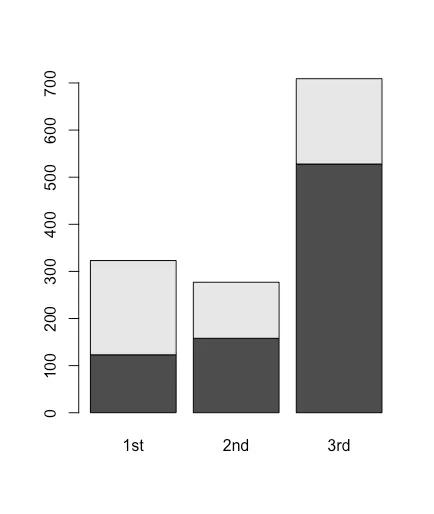```barplot(table(titanic\$survived,titanic\$pclass),
col=c("yellow","dark blue"))```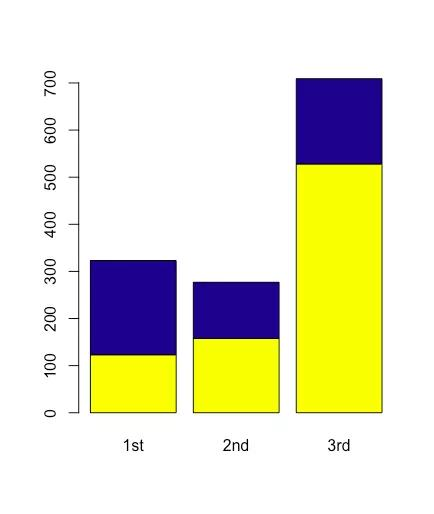```barplot(table(titanic\$survived,titanic\$pclass),
col=c("yellow","dark blue"), beside=T)```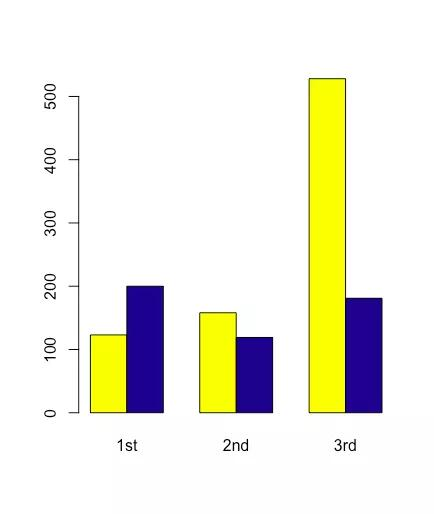```barplot(table(titanic\$survived,titanic\$pclass),
col=c("yellow","dark blue"),
beside=T, legend=T, args.legend=list(x="topleft"),
main="Survival (Pct) by Passenger Class",
xlab="Class",ylab="Count",
ylim=c(0,600))```

legend为设置图例的命令；args.legend为设置图例位置的命令；

main为设置图标题的命令；

xlab、ylab分别为设置x轴和y轴名称的命令；

ylim为设置y轴范围的命令。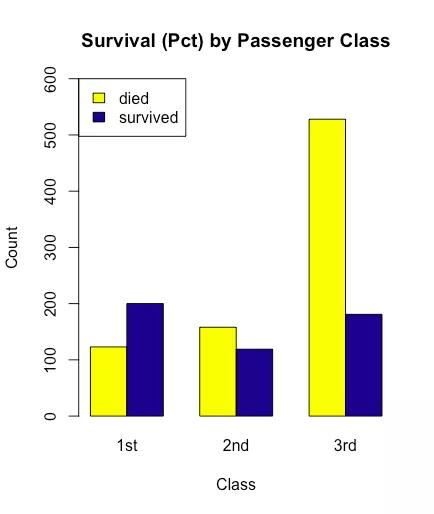`text(c(2,5,8),c(250,250,570),survpct, cex=1.2)`

text()为给图片中增加文字的函数。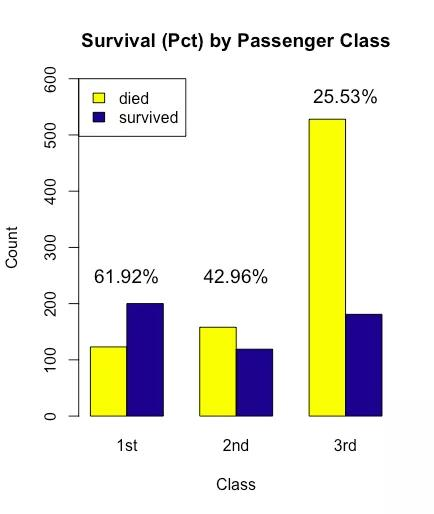```barplot(table(titanic\$survived,titanic\$pclass), col=c("red","blue"),
beside=T, legend=T, args.legend=list(x="topleft"),
main="不同舱位乘客幸存数（率）", xlab="舱位",ylab="人数",family = "SimHei",
ylim=c(0,600))```

family命令需要赋值字体的英文名称，本文将字体设置为"SimHei"，即黑体。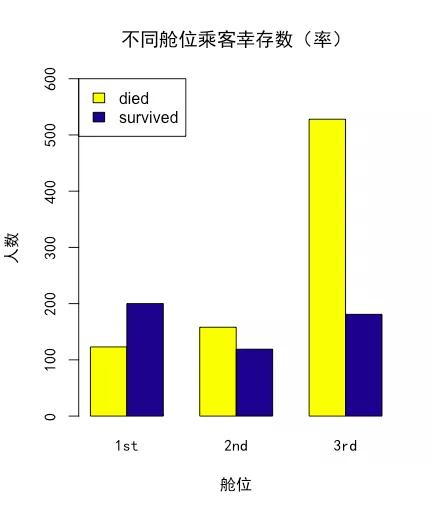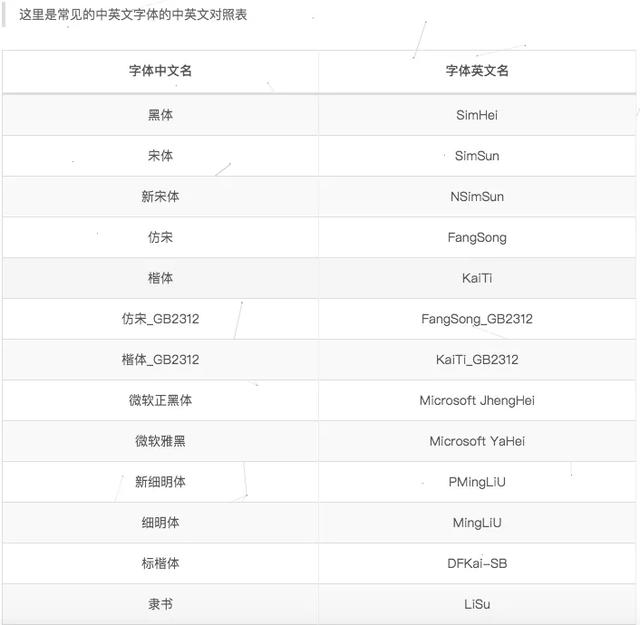OK